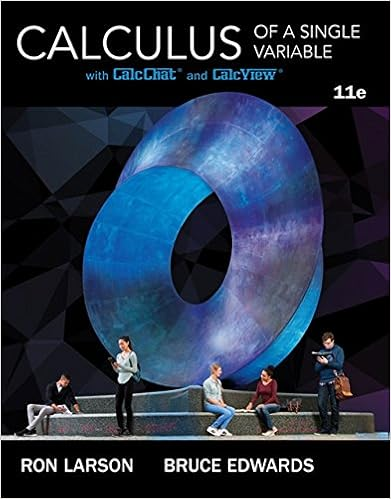# Find an equation of the parabola with vertex 54 and

• Homework Help
• 9

This preview shows page 6 - 8 out of 9 pages.

##### We have textbook solutions for you!
The document you are viewing contains questions related to this textbook.The document you are viewing contains questions related to this textbook.
Chapter 9 / Exercise 49
Calculus of a Single Variable
Edwards/LarsonExpert Verified
12. Find an equation of the parabola with vertex (5,4)and focus (3,4)13. Find the center, foci, vertices, and eccentricity of the ellipse, and sketch its graph.
14. Find an equation of the ellipse with vertices (1,3) and (9,3) and minor axis length 6. 15. Find an equation of the hyperbola with the given characteristics.
16. For the ellipse 2xy14use integration to find (a) Its area, (b) The volume of the solid
Math 150B Unit 7 Written Homework (Due: December 6)1. (Sec. 10.2) Sketch the curve represented by the parametric equations, indicating the direction of the curve, and write the corresponding rectangular equations by eliminating the parameter.
2. Eliminate the parameter and write the corresponding rectangular equation and sketch its graph.
3. Find parametric equations of a line that passes through the points ( 2, 6)
##### We have textbook solutions for you!
The document you are viewing contains questions related to this textbook.The document you are viewing contains questions related to this textbook.
Chapter 9 / Exercise 49
Calculus of a Single Variable
Edwards/LarsonExpert Verified
4. Find two sets of parametric equations for the rectangular equation y6x55. Find the values of at which the curve is not smooth.
(b) 3x3cos, 3y3sin(c) x2cot, 2y2sindx, and find the slope and concavity (if possible) at the given value of the parameter. 6. (Sec. 10.3) Find dydx2Find all points (x,y), if any, of horizontal and vertical tangency to the curve. and
d ydx, and find the slope and concavity (if possible) at the given value of the parameter.
4 7. Find all points (x,y), if any, of horizontal and vertical tangency to the curve.
.
. 9. Find the area of the surface generated by revolving the curve 3xacos, 3yasinon the interval 0   about the x-axis. 10. (Sec. 10.4) Convert the rectangular equation to polar form and sketch its graph.
11. Convert the polar equation to rectangular form and sketch its graph.
12. Find the values of on the interval 02  where there are points of horizontal or vertical tangency to the polar curve.
13. Sketch a graph of the polar equation. For part (b), also find and sketch the tangents at the pole. (a) r2(1sin )
14. Sketch a graph of the polar equation.
15. (Sec. 10.5) Find the area of the polar region.
(b) Common interior of r4sinand 16. Find the arc length of the curve over the interval 02  r2
•••